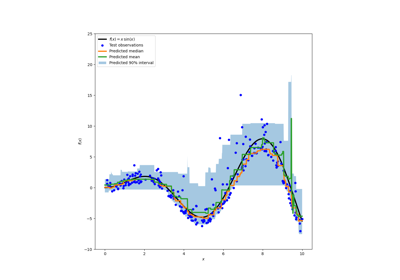# sklearn.metrics.mean_pinball_loss¶

sklearn.metrics.mean_pinball_loss(y_true, y_pred, *, sample_weight=None, alpha=0.5, multioutput='uniform_average')[source]

Pinball loss for quantile regression.

Read more in the User Guide.

Parameters:
y_truearray-like of shape (n_samples,) or (n_samples, n_outputs)

Ground truth (correct) target values.

y_predarray-like of shape (n_samples,) or (n_samples, n_outputs)

Estimated target values.

sample_weightarray-like of shape (n_samples,), default=None

Sample weights.

alphafloat, slope of the pinball loss, default=0.5,

This loss is equivalent to Mean absolute error when alpha=0.5, alpha=0.95 is minimized by estimators of the 95th percentile.

multioutput{‘raw_values’, ‘uniform_average’} or array-like of shape (n_outputs,), default=’uniform_average’

Defines aggregating of multiple output values. Array-like value defines weights used to average errors.

‘raw_values’ :

Returns a full set of errors in case of multioutput input.

‘uniform_average’ :

Errors of all outputs are averaged with uniform weight.

Returns:
lossfloat or ndarray of floats

If multioutput is ‘raw_values’, then mean absolute error is returned for each output separately. If multioutput is ‘uniform_average’ or an ndarray of weights, then the weighted average of all output errors is returned.

The pinball loss output is a non-negative floating point. The best value is 0.0.

Examples

>>> from sklearn.metrics import mean_pinball_loss
>>> y_true = [1, 2, 3]
>>> mean_pinball_loss(y_true, [0, 2, 3], alpha=0.1)
0.03...
>>> mean_pinball_loss(y_true, [1, 2, 4], alpha=0.1)
0.3...
>>> mean_pinball_loss(y_true, [0, 2, 3], alpha=0.9)
0.3...
>>> mean_pinball_loss(y_true, [1, 2, 4], alpha=0.9)
0.03...
>>> mean_pinball_loss(y_true, y_true, alpha=0.1)
0.0
>>> mean_pinball_loss(y_true, y_true, alpha=0.9)
0.0


## Examples using sklearn.metrics.mean_pinball_loss¶Release Highlights for scikit-learn 1.0

Release Highlights for scikit-learn 1.0Prediction Intervals for Gradient Boosting Regression

Prediction Intervals for Gradient Boosting Regression